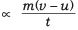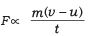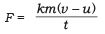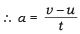Courses

# Long Answers - Force and Laws of Motion, Science, Class 9 Class 9 Notes | EduRev

## Class 9 : Long Answers - Force and Laws of Motion, Science, Class 9 Class 9 Notes | EduRev

The document Long Answers - Force and Laws of Motion, Science, Class 9 Class 9 Notes | EduRev is a part of the Class 9 Course Class 9 Science by VP Classes.
All you need of Class 9 at this link: Class 9

Q1. Explain Newton’s second law of motion and with the help of an example show how it is used in sports.
Ans.
Newton’s second law of motion: The rate of change of momentum of an object is proportional to the applied unbalanced force in the direction of the force.
Let us assume : Object of mass m, is moving along a straight line with an initial velocity ‘u’, It is uniformly accelerated to velocity v in time ‘t’ by the application of force, F throughout the time ‘t’.
Initial momentum of the object = P1 = mu
Final momentum of the object = P2 = mv
The change in momentum ∝ P2 – P1
∝ mv – mu = m (v – u)

The rate of change of momentum∴    Applied force∴     F = k mak = constant of proportionality F = kg m/s2 = Newton

Use of second law of motion in sports: In cricket field, the fielder gradually pulls his hands backward while catching a ball. The fielder catches the ball and gives swing to his hand to increase the time during which the high velocity of the moving ball decreases to zero.
The acceleration of the ball is decreased and therefore the impact of catching the fast moving ball is reduced.
If not done so, then the fast moving ball will exert large force and may hurt the fielder.

Q2. State all 3 Newton’s law of motion. Explain inertia and momentum.
Ans. Newton’s I law of motion:
An object remains in a state of rest or of uniform motion in a straight line unless acted upon by an external unbalanced force.
Newton’s II law of motion: The rate of change of momentum of an object is proportional to the applied unbalanced force in the direction of the force.
Newton’s III law of motion: To every action, there is an equal and opposite reaction and they act on two different bodies.
Inertia: The natural tendency of an object to resist a change in their state of rest or of uniform motion is called inertia.
Momentum: The momentum of an object is the product of its mass andvelocity and has the same direction as that of the velocity.
Its S.I. unit is kg m/s. p = m × v

Q3. Define force. Give its unit and define it. What are different types of forces?
Ans. Force:
It is a push or pull on an object that produces acceleration in the body on which it acts.
A force can do 3 things on a body
(a) It can change the speed of a body.
(b) It can change the direction of motion of a body.
(c) It can change the shape of the body.
The S.I. unit of force is Newton.

Newton: A force of one Newton produces an acceleration of 1 m/s2 on an object of mass 1kg.
1N = 1 kg m/s2 Force = mass × acceleration

Types of forces: (i) Balanced force: When the forces acting on a body from the opposite direction do not change the state of rest or of motion of an object, such forces are called balanced forces.

(ii) Unbalanced force: When two opposite forces acting on a body move a body in the direction of the greater force or change the state of rest, such forces are called as unbalanced force.

(iii) Frictional force: The force that always opposes the motion of object is called force of friction.

Q4. What is inertia? Explain different types of inertia. Give three examples in daily life which shows inertia.
Ans.
Inertia: The natural tendency of an object to resist change in their state of rest or of motion is called inertia.
The mass of an object is a measure of its inertia. Its S.I. unit is kg.
Types of inertia: Inertia of rest: The object at rest will continue to remain at rest unless acted upon by an external unbalanced force.
Inertia of motion: The object in the state of uniform motion will continue to remain in motion with same speed and direction unless it is acted upon by an external unbalanced force.
Three examples of inertia in daily life are:
(i) When we are travelling in a vehicle and sudden brakes are applied we tend to fall forward.
(ii) When we shake the branch of a tree vigorously, leaves fall down.
(iii) If we want to remove the dust from carpet we beat the carpet so that dust fall down.

Q5. Consider the two forces acting on a person standing on the ground; the downward pull of gravity and the upward push of the ground.
(a) Explain whether the forces are equal and opposite.
(b) Explain whether the forces are an action-reaction pair.
Ans.
(a) They are equal and opposite but the forces are not acting on two different bodies, the forces in question are on the same body.
(b) There are two action-reaction pairs which combine to make the effect – gravity provides the Earth-person attraction and the normal perpendicular force provides the Earth-person repulsion it is equal and opposite to prevent the person sinking into the Earth.

Offer running on EduRev: Apply code STAYHOME200 to get INR 200 off on our premium plan EduRev Infinity!

75 docs|14 tests

,

,

,

,

,

,

,

,

,

,

,

,

,

,

,

,

,

,

,

,

,

,

,

,

,

,

,

;